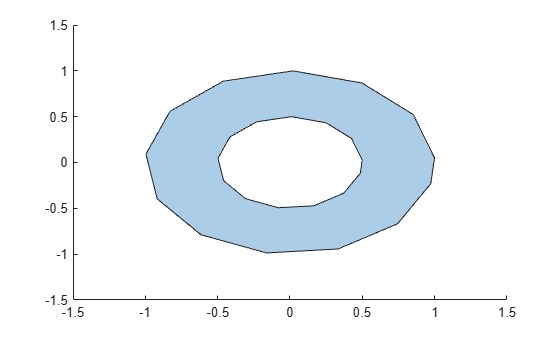# ishole

Determine if `polyshape` boundary is a hole

## Syntax

``TF = ishole(polyin)``
``TF = ishole(polyin,I)``

## Description

example

````TF = ishole(polyin)` returns a logical vector whose elements are 1 (`true`) if the corresponding boundary of `polyin` is a hole.```
````TF = ishole(polyin,I)` returns a logical vector corresponding to the boundaries of `polyin` indexed by `I`. `TF` is the same length as `I`.```

## Examples

collapse all

Create a polygon containing one solid region and one hole, and determine which region is a hole.

```t = 0.05:0.5:2*pi; x1 = cos(t); y1 = sin(t); x2 = 0.5*cos(t); y2 = 0.5*sin(t); polyin = polyshape({x1,x2},{y1,y2})```
```polyin = polyshape with properties: Vertices: [27x2 double] NumRegions: 1 NumHoles: 1 ```
`plot(polyin)``TF = ishole(polyin)`
```TF = 2x1 logical array 0 1 ```

To query one boundary at a time, use the boundary index as a second argument.

`TF = ishole(polyin,2)`
```TF = logical 1 ```

## Input Arguments

collapse all

Input `polyshape`, specified as a scalar.

Data Types: `polyshape`

Boundary index, specified as a scalar integer or vector of integers. Each element of `I` corresponds to a single boundary of the input `polyshape`.

Data Types: `double` | `single` | `int8` | `int16` | `int32` | `int64` | `uint8` | `uint16` | `uint32` | `uint64`